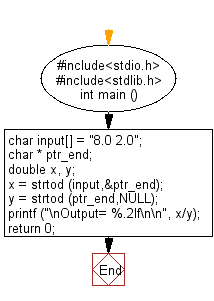﻿ C Program: Convert a string to double - w3resource

# C Exercises: Convert a string to double

## C Variable Type : Exercise-4 with Solution

Write a C program to convert a string to a double.

Sample Solution:

C Code:

``````#include<stdio.h>      // Include the standard input/output header file.
#include<stdlib.h>     // Include the standard library header file.

int main ()          // Start of the main function.
{
char input[] = "8.0 2.0";  // Declare a character array 'input' and initialize it with "8.0 2.0".
char * ptr_end;           // Declare a pointer to char 'ptr_end'.

double x, y;              // Declare two double variables 'x' and 'y'.

x = strtod (input,&ptr_end);   // Convert the first part of 'input' to a double, store in 'x', and update 'ptr_end'.
y = strtod (ptr_end,NULL);     // Convert the remaining part of 'input' to a double, store in 'y'.

printf ("\nOutput= %.2lf\n\n", x/y);   // Print the result of x/y with two decimal places.

return 0;   // Return 0 to indicate successful execution of the program.
}   // End of the main function.
```
```

Sample Output:

```Output= 4.00
```

Flowchart:C Programming Code Editor:

What is the difficulty level of this exercise?

Test your Programming skills with w3resource's quiz.

﻿